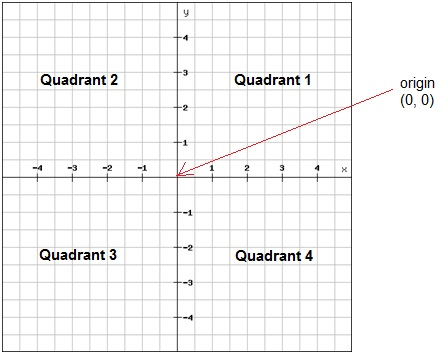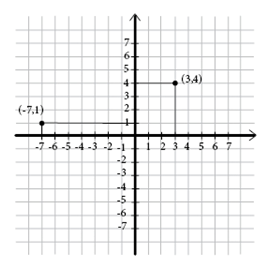# Coordinate system and ordered pairs

A coordinate system is a two-dimensional number line, for example, two perpendicular number lines or axes.

This is a typical coordinate system:The horizontal axis is called the x-axis and the vertical axis is called the y-axis

The center of the coordinate system (where the lines intersect) is called the origin. The axes intersect when both x and y are zero. The coordinates of the origin are (0, 0).

An ordered pair contains the coordinates of one point in the coordinate system. A point is named by its ordered pair of the form of (x, y). The first number corresponds to the x-coordinate and the second to the y-coordinate.

To graph a point, you draw a dot at the coordinates that corresponds to the ordered pair. It's always a good idea to start at the origin. The x-coordinate tells you how many steps you have to take to the right (positive) or left (negative) on the x-axis. And the y-coordinate tells you have many steps to move up (positive) or down (negative) on the y-axis.

ExampleThe ordered pair (3, 4) is found in the coordinate system when you move 3 steps to the right on the x-axis and 4 steps upwards on the y-axis.

The ordered pair (-7, 1) is found in the coordinate system when you move 7 steps to the left on the x-axis and 1 step upwards on the y-axis.

To find out the coordinates of a point in the coordinate system you do the opposite. Begin at the point and follow a vertical line either up or down to the x-axis. There is your x-coordinate. And then do the same but following a horizontal line to find the y-coordinate.

## Video lesson

Draw the following ordered pairs in a coordinate plane (0, 0) (3, 2) (0, 4) (3, 6) (6, 9) (4, 0)